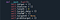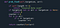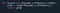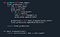# Can you make a better mousetrap?

In the process of learning more and better coding skills I have been tasked with doing the unthinkable… making a predictive modeling algorithm mimicking a Naive Bayesian Classifier.

The formula for Bayesian probability is not complicated, it only asks for 3 values.

I won’t go into too much detail but each time you see a big “P” that’s a probability. The probability you want is the one on the left side of the equal sign and everything else is what you need to supply the formula. To make a prediction with this though requires that all the data you take in be processed through this formula.

The Set Up

Getting the probability of each unique value is an undertaking when drawing up the plans but eventually a simple technique is developed by counting the instances occurring and dividing those by the total volume of data for each section or category. This is thankfully done by the computer as most of the time the data used for these predictions comes as a massive data-frame which must be broken into individual arrays as categories of information.

The really complicated part is trying to get the value shown in the formula as “P(B|A)” which translates to the probability of A being true given that B is true. What this turns into is trying to run calculation of probability for every unique value of the prediction target against every unique value of the data being used to make your prediction.

Organization

To save time I used dictionaries to reference with the different unique values as the keys and saved them for each category of data used for predictions. This allowed me to run through each dictionary and perform this probability equation based on the saved values then save the highest probability as my predicted target value for each unique value in the predictive data. This works by saying if I’m trying to predict the color of a vehicle and 60% of cars are Blue and 70% of trucks are Red I’m going to just go with Red if the vehicle is a truck because it’s the best option based on probability.“self.target” is the array of data that we are training the model to predict.

Here is where I set up all the main variables. The “self.target” is the array of data that we are using as an example to train the model on so we can make predictions. The “self.features” is the 2-D array with many types of data that are the matching pieces of data that the model connects to the example “self.target”. The variable “self.targetUS” is an empty value which will be filled with a set of unique values from “self.target”. “self.target_data” is a dictionary which is filled with the unique values of the “self.targetUS” set as keys connected to the probability values of each of those unique values. The slightly more confusing “self.TSGFWP” and “self.probGtarget” are dictionaries which I will fill with probabilities of Target Set Given Feature With Probability which means the probability of the Target values given a feature value, and the probability given target which is the probability of a feature value given a target value accordingly. Both of these are the last values filled as they require the most functions to run.

This is not precise but I also go by the most commonly predicted value in an entire row of data including each category of data, so it’s more of a democratic system of prediction based on probability. The algorithm then returns a list of predicted values (final_prediction based on the most commonly predicted value per each row of data values.

Troubles

There are a few likely issues with this simplified version.

1. The model is using the highest probability response so any one data value will only give one response every time regardless of the other data values. This is by the nature of the name “Naive” which means each of the values are considered individually and not in consideration to the other data values. But going by the previous example that means every time it sees the vehicle is a truck it will always say the color is Red no matter what, which can lead to responses that have poor correlation.
2. To use this hand-made model you will need to have very meaningful data to train with or it will make bad predictions based on unimportant information. This model however doesn’t need the data to be made into numbers and will run no matter the data type save for None type.
3. You should also have clean data as unfortunately the model doesn’t work with NAN or empty values. If your data isn’t clean, then you should use an encoder which will convert the empty value into a number.

The Take Away

I learned a lot about what goes into the tools I’ve used in data-science and it gives me an appreciation for how much coding skill is required to create any one of them. Given several months and a budget I can see how a much better model could be formed with a small team.

Some of my functions in the Naive Bayesian Categorical Classifier classMy function to find probability for each unique value in an array.My function to perform the Bayesian probability.My predict attribute and it’s helper function to find winning predictions.